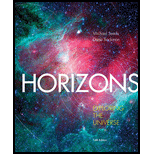Chapter 8, Problem 12RQHorizons: Exploring the Universe (...

14th Edition
Michael A. Seeds + 1 other
ISBN: 9781305960961

Solutions

Chapter
SectionHorizons: Exploring the Universe (...

14th Edition
Michael A. Seeds + 1 other
ISBN: 9781305960961
Textbook Problem

Why does the orbital period of a binary star depend on its mass?

To determine

Why does the orbital period of a binary star depend on its mass?

Explanation

The binary star system is a system in which two stars rotate about a system center of mass due to the force of gravitation between two stars.

The force of gravitation is given as

F=Gm1m2r2

Now if one star is massive than its

Still sussing out bartleby?

Check out a sample textbook solution.

See a sample solution

The Solution to Your Study Problems

Bartleby provides explanations to thousands of textbook problems written by our experts, many with advanced degrees!

Get Started